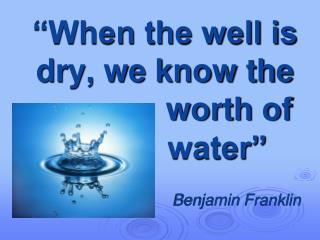DownloadDownload Presentation“When the well is dry, we know the worth of water”

# “When the well is dry, we know the worth of water”

Download Presentation## “When the well is dry, we know the worth of water”

- - - - - - - - - - - - - - - - - - - - - - - - - - - E N D - - - - - - - - - - - - - - - - - - - - - - - - - - -
##### Presentation Transcript

1. “When the well is dry, we know the worth of water” Benjamin Franklin

2. Mineral water (integrated mathematic-chemistry lesson)

3. To explore general mineralization of mineral water. To measure qualitative components of mineral water “Vytautas”. To learn to solve integrated mathematics-chemistry tasks. to get acquainted with variety of mineral waters of Lithuania. The aim of the lesson: The goals of the lesson:

4. The classification of mineral water • Mineral waters wich have a lot of minerals: the concentration of minerals > 1500mg/l. • Examples: • “Rasa” • “Vytautas” • “Naleczovanka” • “Augustovianka” • Mineralwaterswichhaveveryfewminerals: theconcentrationofminerals ≤ 50mg/l. • Examples: • “Neptūnas” • “Rytas” • “Perrier” • “Vittel” • Mineral waters wich have few minerals: the concentration of minerals ≤ 500mg/l.

5. Answers of group chemistry tasks: Group 1 : Ag+(aq) + Cl-(aq) → AgCl(s)Group 2 : Ca2+(aq) + CO32-(aq) → CaCO3(s)Group 3 : Ba2+(aq) + SO42-(aq) → BaSO4(s)Group 4 : H+(aq) + HCO3-(aq) → CO2(g) + H2O(l)

6. C = • 534 mg/l · 1l = 534 mg • = 0,534 g • n(Ca2+)= = 0,013mol • C = • Ca2+(aq) + Na2CO3(aq) → CaCO3(s) + 2Na+(aq) • n(Ca2+) = 0,013 mol • n(Na2CO3) = 0,013 mol • C = • V= = 0,026l

7. “Vytautas” • 3437mg/l · 1l = 3437mg • m(Cl-) = = 3,437g • w(Cl-) = · 100% = 47,98% • “Perrier” • 21,5mg/l ·1l = 21,5mg • m(Cl-) = • w(Cl-) = · 100% = 3,56%

8. 1. Let us assume, that: x is the number of small bottles (0,5l), y isthe number of large bottles (1,5l). Make an equation system: x+y = 36 0,5x + 1,5y = 42 x = 36 – y 0,5x + 1,5y = 42 0,5 · (36 – y) + 1,5y = 42 18 – 0,5y + 1,5y = 42 y = 42 – 18 y = 24 x = 36 – 24 = 12 The answer: there are 12 small bottles (0,5l) and 24 large bottles (1,5l)

9. Let us assume, that: x is the number of small bottles (0,5l) of mineral water “Vytautas” y is the number of small bottles (0,5l) of mineral water “Rasa” Make an equation system: x + y = 12 x = y + 2 y + 2 + y = 12 2y = 12 – 2 2y = 10 / ÷2 y = 5 x = 5 + 2 = 7 Let us assume, that: t is the number of large bottles (1,5l) of mineral water “Tiche” z is the number of large bottles (1,5l) of mineral water “Vytautas” Make an equation system: t + z = 24 t = 3z 3z + z = 24 4z = 24 / ÷4 z = 6 t = 3 · 6 = 18 2.

10. 2. 3. Count the praise: 5 · 1,39 + 7 · 1,49 + 6 · 2,29 + 18 · 2,09 = 68,74 The answer: 68,74 Litas Convert to Euros: 68,74 ÷ 3,4528 ≈ 19,9 Convert to Zlotys: 68,74 ÷ 0,81765 ≈ 84,07

11. Conclusion Mineral water is underwater which contains minerals and has curative features; 1 litre of mineral water should contain not less than 1gr of minerals; The composition of salts in mineral water should be constant (stable); Ionic composition of mineral water and the concentration of ions are indicated on the bottle sticker (label).